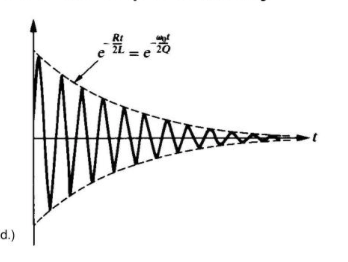# Measuring 10Vpp with Arduino ADC without attenuation

This is possible.
Full wave rectifier circuit (Rail to rail op-amps).
Op-amp supply voltage 2 x the Arduino supply voltage.

I am using a small circuit a "full wave" rectifier circuit to change the sine wave signal from a signal going from a positive value to a negativ value oround zero to a signal going from zero to the peak value of the signal.
By using an op-amp designed as a rail-to-rail op-amp the specifications for the signal handling can be as high as a peak - peak value of 9.8V / peak value 4.9V of the measured signal.
To handle this signal the op-amp is driven by a supply volte of +/- 5V.
This is not a problem for the Arduino analog input because the rectifier ensures the output voltage of the circuit is never less then zero and the op-amp will be clipping peak signals higher 5V.
The calculation also run faster on Arduino, because the Arduino "see" the signal at double frequency, which means the sample time can be reduced up to 50% or the measuring presicion can be significant improved.

This can be used for measuring:
• RMS
• Peak-Peak
• Peak
• Average
voltage of a sine signal

This is a code used for measuring the rectified sine voltage.

``````  // --- sub variables --------------------------------------
int uMax = 0, Uin = 0, SampleTime = 50;
unsigned long startMillis = millis();
// --- sampling -------------------------------------------
while (millis() - startMillis < SampleTime){
uMax = max(uMax, Uin);
}
// --- voltage calculation -------------------------------
mVrms = (uMax * 5.0 * 1000 / 1024) / sqrt(2);
mVpp  = (uMax * 5.0 * 1000 / 1024) * 2;
mVp   = (uMax * 5.0 * 1000 / 1024);
mVavg = (uMax * 5.0 * 1000 / 1024) * (2 / 3.14);
``````

edit: this is the idea of the use of a full wave rectifier for Arduino ADC

That's an interesting idea - for instance you can measure rms voltage from a (precision) rectified version of a signal.

However the precision rectifier circuit needs 0.6V headroom for the forward biased diodes, so a +/-5V signal can only deal with a max of 8.8V peak2peak I reckon.

Can I catch 5 peaks of this his signal using this program ?I am actually using a "full wave" precision rectifier circuit. The circuit do not need the 0.6V headroom. The peak to peak voltage is only depending on the op-amp rail to rail specifications.

I would be happy to share the circuit if I could find out how to upload pictures to the forum.

79galinakorczak
No, this is not the scope of the suggestion, sorry.

If its the same as the one here, it needs a diode-drop headroom: https://circuitdigest.com/electronic-circuits/half-wave-and-full-wave-precision-rectifier-circuit-using-op-amp

MarkT
My circuit is different of the link you provided.
Spice simulation and measurement of the circuit:
Op amp: LT 1638
Measured
U supply: ±5V
Upp: 9,8V (before clipping).

This is the Spice simulation result of the suggeted circuit for Arduino ADC measurement of a sine signal.

That circuit is more sophisticated, but it still saturates on a +5V input - it will work with -5V input, but at +5V input U2 has to output -5.6V to maintain the feedback loop (R4 has no current as D2 is reverse biased)

Also a "rail to rail" op amp will only provide "rail to rail" output voltages if the output is unloaded.
In the diagram the +input to U2 is grounded - so the - input becomes a "virtual ground" and the output of U1 is effectively loaded by 10k to ground.

Of course Spice will assume Rout for U1 is zero unless you include a resistor to simulate the output resisitance.

This topic was automatically closed 120 days after the last reply. New replies are no longer allowed.